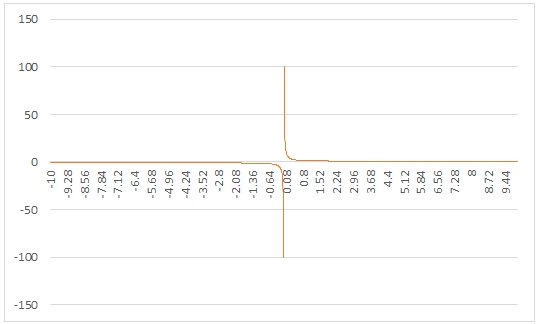# SQL Server COTH function

COTH
Updated: 25 Apr 2017

Use the scalar function COTH to calculate the hyperbolic cotangent of a hyperbolic angle.

Syntax
SELECT [wct].[COTH] (
<@Z, float,>)
Arguments
 Input Name Description @Z The number of interest. @Z must be of type float or of a type that implicitly converts to float.
Return Type
float
Remarks
Examples
Example #1
SELECT
wct.COTH(2) as COTH

This produces the following result.

Example #2

The following SQL can be run and the results pasted into Excel to graph the results of the function in the range -10, 10.

SELECT
SeriesValue as z
,wct.COTH(Seriesvalue) as COTH
FROM
wct.SERIESFLOAT(-10,10,.01,NULL,NULL)

Here's the graph of the resultsCopyright 2008-2023 Westclintech LLC         Privacy Policy        Terms of Service